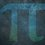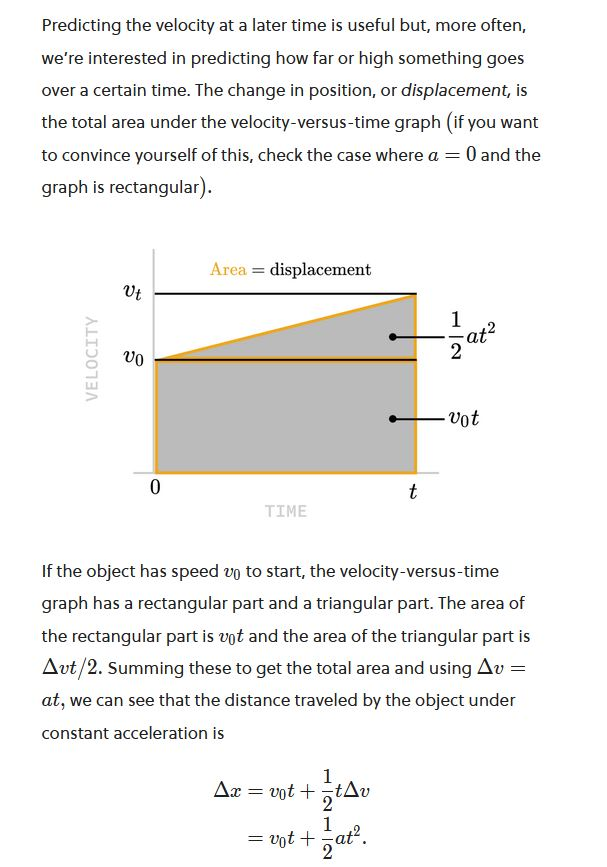# Recurring Proof Note $1$

I'll prove that:

$y = 0.\overline{x_1}x_2 =$$\frac{x_1}{9}$

Let:

$y = 0.\overline{x_1}x_2$

$10y = x_1.\overline{x_1}x_2$

$10y - y = 9y$

$x_1.\overline{x_1}x_2 - 0.\overline{x_1}x_2 = x_1$

$9y = x_1$

$\frac{9y}{9}$ $=$ $\frac{x_1}{9}$

$y =$$\frac{x_1}{9}$

Therefore, $y = 0.\overline{x_1}x_2 =$$\frac{x_1}{9}$Note by Yajat Shamji
1 year ago

This discussion board is a place to discuss our Daily Challenges and the math and science related to those challenges. Explanations are more than just a solution — they should explain the steps and thinking strategies that you used to obtain the solution. Comments should further the discussion of math and science.

When posting on Brilliant:

• Use the emojis to react to an explanation, whether you're congratulating a job well done , or just really confused .
• Ask specific questions about the challenge or the steps in somebody's explanation. Well-posed questions can add a lot to the discussion, but posting "I don't understand!" doesn't help anyone.
• Try to contribute something new to the discussion, whether it is an extension, generalization or other idea related to the challenge.

MarkdownAppears as
*italics* or _italics_ italics
**bold** or __bold__ bold
- bulleted- list
• bulleted
• list
1. numbered2. list
1. numbered
2. list
Note: you must add a full line of space before and after lists for them to show up correctly
paragraph 1paragraph 2

paragraph 1

paragraph 2

[example link](https://brilliant.org)example link
> This is a quote
This is a quote
    # I indented these lines
# 4 spaces, and now they show
# up as a code block.

print "hello world"
# I indented these lines
# 4 spaces, and now they show
# up as a code block.

print "hello world"
MathAppears as
Remember to wrap math in $$ ... $$ or $ ... $ to ensure proper formatting.
2 \times 3 $2 \times 3$
2^{34} $2^{34}$
a_{i-1} $a_{i-1}$
\frac{2}{3} $\frac{2}{3}$
\sqrt{2} $\sqrt{2}$
\sum_{i=1}^3 $\sum_{i=1}^3$
\sin \theta $\sin \theta$
\boxed{123} $\boxed{123}$

Sort by:

@Zakir Husain, what do you think of my first proof?

- 1 year ago

What first proof?

- 1 year ago

The one above?

- 1 year ago

A result for general case: Consider a number $l=0.\overline{x_1x_2x_3...x_n}$ $10^nl=x_1x_2x_3...x_n\large{.}\overline{x_1x_2x_3...x_n}$ $\Rightarrow 10^nl-l={x_1x_2x_3...x_n}$ $(10^n-1)l=x_1x_2x_3...x_n$ $\boxed{l=\dfrac{x_1x_2x_3...x_n}{10^n-1}}$ $\boxed{0.\overline{x_1x_2x_3...x_n}=\dfrac{x_1x_2x_3...x_n}{10^n-1}}$

Note :

• $x_1x_2$ act as number with digits $x_1,x_2$ for example if $x_1=5$ and $x_2=8\Rightarrow x_1x_2=58$ dont confuse ($x_1x_2\cancel{=}x_1\times x_2$)

• $0.\overline{a}=0.aaaaa...$

- 1 year ago

@Yajat Shamji - Here?

Derivation - $v^{2} - u^{2} = 2aS$

We know that $\dfrac{v - u}{t} = a$ because $v-u$ is the change in velocity, and dividing by time we just get change in velocity over time, which is nothing but acceleration.

We also know that $S = ut + \dfrac{1}{2}at^{2}$ as it has been proved in the preface of a kinematics problem. Here is the image of the proof -Now we can use these equations to prove the one we used to solve the problem.

In the equation, we don't have time, so let's find the value of time, and then substitute.

$\dfrac{v - u}{t} = a$ can be changed to $\dfrac{v - u}{a} = t$ by some transposition. Let's substitute this in the other equation now.

\begin{aligned} S &= ut + \dfrac{1}{2}at^{2} \\ S &= u\dfrac{v-u}{a} + \dfrac{1}{2}a\dfrac{v-u}{a})^{2} \\ S &= \dfrac{uv-u^{2}}{a} + \dfrac{v^{2}-2uv + u^{2}}{2a} \\ S &= \dfrac{2uv-2u^{2}}{2a} + \dfrac{v^{2}-2uv + u^{2}}{2a} \\ 2aS &= 2uv-2u^{2} + v^{2}-2uv + u^{2} \\ 2aS &= v^{2} - u^{2} \\ v^{2} - u^{2} &= 2aS \end{aligned}

Hence Proved :)

- 7 months, 4 weeks ago

@Percy Jackson $\int_{A}^{B} \vec{a} \cdot d\vec{s} = \int_{A}^{B} \frac{d\vec{v}}{dt} \cdot d\vec{s} = \int_{A}^{B} d\vec{v} \cdot \vec{v}$ $= { {}} \int_{A}^{B} v_x dv_x + {{{ }}} \int_{A}^{B} v_y dv_y + { { }}\int_{A}^{B} v_z dv_z$ $=\frac{1}{2}(v^2_{x,B} - v^2_{x,A} + v^2_{y,B} - v^2_{y,A} + v^2_{z,B} - v^2_{z,A}) = \frac{1}{2}(\vec{v_{B}} \cdot \vec{v_{B}} - \vec{v_{A}} \cdot \vec{v_{A}})$ $\Rightarrow 2 \int_{A}^{B} \vec{a} \cdot d\vec{s} = \vec{v_{B}} \cdot \vec{v_{B}} - \vec{v_{A}} \cdot \vec{v_{A}}$ For constant $\vec{a}$ $2\vec{a}(\vec{s}_B - \vec{s}_A) = \vec{v_{B}} \cdot \vec{v_{B}} - \vec{v_{A}} \cdot \vec{v_{A}}$

- 7 months, 4 weeks ago

That's fine.

- 7 months, 3 weeks ago